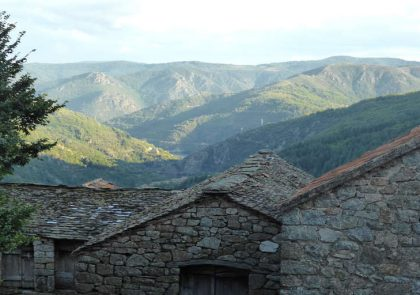# Blog

We provide practical tutorials on data mining, visualization and statistics for decision making.This article describes how to remove legend from a plot created using the ggplot2 package. You will learn how to: Hide the entire legend to create a ggplot with no legend. Remove the legend for a specific aesthetic. Prerequisites Load required packages and set...

#### How to Remove Legend from a GGPlot

This article describes how to remove legend from a plot created using the ggplot2 package. You will learn how to: Hide the entire legend to create a ggplot with no legend. Remove the legend for a specific aesthetic. Prerequisites Load required packages and set...This article describes how to change ggplot legend size. You will learn how to modify the legend title and text size. Prerequisites Load required packages and set the theme function theme_minimal() as the default theme: library(ggplot2) theme_set(theme_minimal()) Basic plot Start by creating a box...

#### How to Change GGPlot Legend Size

This article describes how to change ggplot legend size. You will learn how to modify the legend title and text size. Prerequisites Load required packages and set the theme function theme_minimal() as the default theme: library(ggplot2) theme_set(theme_minimal()) Basic plot Start by creating a box...This tutorial shows how to add title to a ggplot. The function labs() is used. Prerequisites Load required packages and set the theme function theme_minimal() as the default theme: library(ggplot2) # For data visualization theme_set(theme_minimal()) Add title, subtitle and caption # Default plot library(ggplot2)...

#### How to Add Title to a GGPlot

This tutorial shows how to add title to a ggplot. The function labs() is used. Prerequisites Load required packages and set the theme function theme_minimal() as the default theme: library(ggplot2) # For data visualization theme_set(theme_minimal()) Add title, subtitle and caption # Default plot library(ggplot2)...This tutorial describes how to create a ggplot stacked bar chart. You will also learn how to add labels to a stacked bar plot. Prerequisites Load required packages and set the theme function theme_minimal() as the default theme: library(dplyr) # For data manipulation library(ggplot2)...

#### How to Create a GGPlot Stacked Bar Chart

This tutorial describes how to create a ggplot stacked bar chart. You will also learn how to add labels to a stacked bar plot. Prerequisites Load required packages and set the theme function theme_minimal() as the default theme: library(dplyr) # For data manipulation library(ggplot2)...This tutorial describes how to create a ggplot with multiple lines. Load ggplot2 package Load ggplot2 and set the default theme: library(ggplot2) theme_set(theme_minimal()) Data The US economics time series datasets are used. This is a data frame with 478 rows and 6 variables. head(economics)...

#### How to Create a GGPlot with Multiple Lines

This tutorial describes how to create a ggplot with multiple lines. Load ggplot2 package Load ggplot2 and set the default theme: library(ggplot2) theme_set(theme_minimal()) Data The US economics time series datasets are used. This is a data frame with 478 rows and 6 variables. head(economics)...This R graphics tutorial describes how to change line types in R for plots created using either the R base plotting functions or the ggplot2 package. In R base plot functions, the options lty and lwd are used to specify the line type and...

#### Line Types in R: The Ultimate Guide for R Base Plot and GGPLOT

This R graphics tutorial describes how to change line types in R for plots created using either the R base plotting functions or the ggplot2 package. In R base plot functions, the options lty and lwd are used to specify the line type and...This article describes how to change a ggplot point shapes. You will learn how to: Change ggplot point shape values. In ggplot, point shapes can be specified in the function geom_point(). Key arguments include: shape: numeric values as pch for setting plotting points shapes....

#### GGPLOT Point Shapes Best Tips

This article describes how to change a ggplot point shapes. You will learn how to: Change ggplot point shape values. In ggplot, point shapes can be specified in the function geom_point(). Key arguments include: shape: numeric values as pch for setting plotting points shapes....This article describes the different pch in R for modifying the point symbols of an R base plot. The option pch is used to specify point symbols in the functions plot() and lines(). In this tutorial, you’ll learn how to: Display easily the list...

#### PCH in R Best Tips

This article describes the different pch in R for modifying the point symbols of an R base plot. The option pch is used to specify point symbols in the functions plot() and lines(). In this tutorial, you’ll learn how to: Display easily the list...This article describes how to simulate colorblindness vision in production-ready R figures using the colorblinr package. We’ll also present some colorblind-friendly palette. In some populations, up to 10% of men have color vision deficiencies (cvd). The R package colorblindr can be used to simulate...

#### How to Stimulate Colorblindness Vision in R Figures

This article describes how to simulate colorblindness vision in production-ready R figures using the colorblinr package. We’ll also present some colorblind-friendly palette. In some populations, up to 10% of men have color vision deficiencies (cvd). The R package colorblindr can be used to simulate...This tutorial describes how to generate color palettes in R. Color is crucial for elegant data visualization. You will learn the basics for using the power of color and, we describe an R package and an online tool for generating beautiful color schemes. Contents:...

#### How to Create Beautiful Color Palettes in R

This tutorial describes how to generate color palettes in R. Color is crucial for elegant data visualization. You will learn the basics for using the power of color and, we describe an R package and an online tool for generating beautiful color schemes. Contents:...